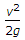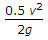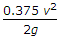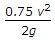# Mechanical Engineering - Hydraulics and Fluid Mechanics

### Exercise :: Hydraulics and Fluid Mechanics - Section 3

16.

The velocity corresponding to Reynold number of 2000 is called

 A. sub-sonic velocity B. super-sonic velocity C. lower critical velocity D. higher critical velocity

Answer: Option C

Explanation:

No answer description available for this question. Let us discuss.

17.

The specific weight of water in S.I. units is taken as

 A. 9.81 kN/m3 B. 9.81 x 103 kN/m3 C. 9.81 x 10-6 N/mm3 D. any one of these

Answer: Option A

Explanation:

No answer description available for this question. Let us discuss.

18.

The loss of head at exit of a pipe is

 A.B.C.D.Answer: Option A

Explanation:

No answer description available for this question. Let us discuss.

19.

The flow in a pipe is laminar, when Reynold number is less than 2000.

 A. True B. False

Answer: Option A

Explanation:

No answer description available for this question. Let us discuss.

20.

The power transmitted through the pipe is maximum when the head lost due to friction is equal to

 A. one-fourth of the total supply head B. one-third of the total supply head C. one-half of the total supply head D. two-third of the total supply head

Answer: Option B

Explanation:

No answer description available for this question. Let us discuss.

#### Current Affairs 2021

Interview Questions and Answers